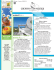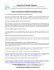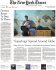# Math 321- Spring 2013 - Exam2 Sample

## Transcription

Math 321- Spring 2013 - Exam2 Sample
```Math 321- Spring 2013 - Exam2 Sample
Instructor: Dr. Francesco Strazzullo
Name
Instructions. Technology and notes (including the formula sheets from our book) are allowed on this exam. Each
problem is worth 10 points. If you use notes or formula sheets, make a reference. When using technology describe
which commands (or keys typed) you used or print out your worksheet.
1. A bacteria culture starts with 350 bacteria and in 75 minutes contains 700 bacteria.
(a) Use a natural exponential growth ODE to model this culture.
(b) How many hours does it take to reach 14000 bacteria?
(
2. Check if the function y = 3x − 1 + 2e−x is a solution of the IVP
1
y 00 − y 0 − 2y = 5 − 6x
y(0) = −1,
y 0 (0) = 1
.
3. Find the orthogonal trajectories of the family of curves y = kx2 . Then draw several members of each family on
the same coordinate plane. (You can attach a printout or upload it in EagleWeb.)
2
4. Suppose a population growth is modeled by the logistic differential equation with the carrying capacity 2000
and the relative growth rate k = 0.06 per year.
(a) Express the logistic equation.
(b) Express the general solution.
(c) Express the particular solution for which P (0) = 500.
3
5. A phase portrait of a predator-prey system is given below in which F represents the population of foxes (in
thousands) and R the population of rabbits (in thousands).
(a) Referring to the graph, what is a reasonable non-zero equilibrium solution for the system?
(b) Write down a possible system of differential equations which could have been used to produce the given
graph.
4
6. Consider the following predator-prey system where x and y are in millions of creatures and t represents time in
years:

dx


= 2x − xy
dt

 dy = −4y + xy
dt
(a) Show that (4, 2) is the nonzero equilibrium solution.
dy
(b) Find an expression for
.
dx
(c) The direction field for the differential equation at point (6b) is given below. Locate (4, 2) on the graph and
sketch a rough phase trajectory through P indicated in the graph.
5
```

### Sir M. Visvesvaraya senior scientist`s state award for science and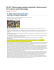### BC Calculus 6.4 Practice worksheet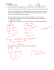### UNWANTED HOUSEGUESTS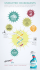### Привет! (Privyet!) Have a pencil/pen and journal ready!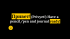###     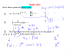### Epulopiscium fishelsoni - Academic lab pages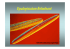### Dry Air Storage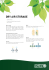### 8-2 Enrich: Identifying Bacteria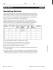### The hub of diseases-converted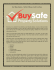### File - math with mrs. Alligood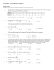### HW6 due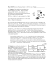### , Keeping healthy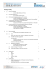### MTH 234 Groupwork 5 1. Salmonella bacteria, found on almost all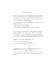### Problem 1 Consider the Euler equation t x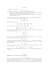### Fantastic Foamy Fountain.pages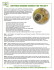### alarm force fear factor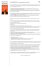### Sample Submission Form Pharmaceutical Microbiology Aemtek No.: Project Information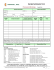### as PDF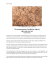### 3.1 exponential growth worksheet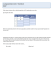### August